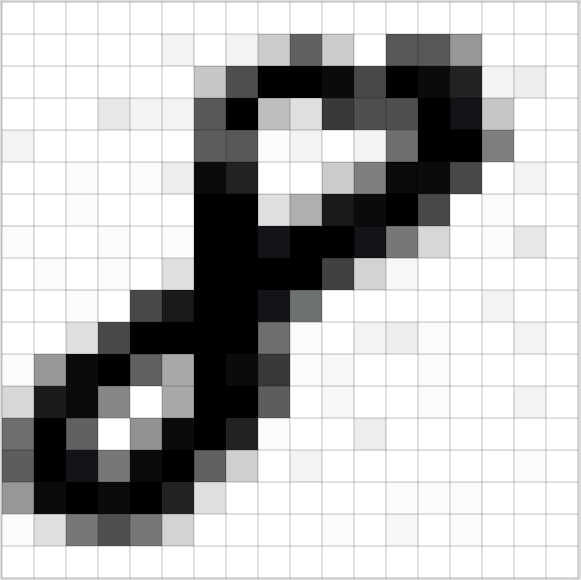# What are Neural Networks

Written by Thomas Tumiel

##### Why is this Important:
Neural networks are the backbone to vast amounts of machine learning breakthroughs. Knowing how they work is not just important, but vital to becoming a machine learning practitioner.

Neural networks may seem like a black box to the uninitiated. In this tutorial, we will look into how a neural network (and most modern machine learning) works.

## Introduction

A neural network is a mathematical function: it takes an input and generates an output. You might be used to simpler functions such as $$y=ax+b$$, a straight line, that converts an input $$x$$ to an output $$y$$. Very similarly, neural networks transform their input into an output.

## Forward Propagation

When you convert an input x into an output y in a neural network, this is called forward propagation. Most of the time, we want our network to predict something useful such as whether an image contains a cat. We can do this by making the input the image in question and the output a number between 0 and 1 - 0 for no cat, 1 for cat. The input image is actually a matrix of numbers that has the same width and height as the image. This is because the numbers represent the colours of the pixel at that point in the image.Image from here

So we take each of these input numbers in the image and according to some function that our neural network decides on, we generate an output deciding if there is a cat or not. That is, we propagate the input image forward through the neural network in order to generate an output prediction of cat or not.

## Backward Propagation

But how does the neural network learn that function? It learns it using data. If we have 100 images of cats and 100 images without cats, then we can “train” our neural network to “recognise” what a cat might look like in terms of the underlying pattern of data. And this is how it works at a high level:

First, the image is forward propagated to get a prediction of what the image is (cat or not). In the beginning, before the network is trained, the predictions are just random. Then we compare this prediction to our true label of the image (the image is, say, a cat). By comparing the prediction to the actual value, we get a loss. A loss tells us how far off our network is from where it should be.Photo by Erik-Jan Leusink

For example, say our network predicted that this image was not a cat by labeling it as 0.32, which is closer to 0 and thus classified as “not cat”. The correct prediction is that it is a cat with true label 1. The loss simply looks something like 1-0.32 = 0.68. If our prediction gets worse (closer to 0 in this case), the loss increases, if our prediction gets better, our loss decreases.

Now once we have the loss, we want to find out how to minimize it. This process of minimization is called optimization.

### Optimization

Optimization is the process of adjusting the neural network parameters in order to minimize the loss. Specifically, we want to iteratively improve our predictions that cats are in fact cats.

Blindfolded hiker analogy: One analogy that you may find helpful going forward is to think of yourself as hiking on a hilly terrain with a blindfold on, and trying to reach the bottom. You try to feel around you to judge which way is going down the most and move in that direction, constantly checking that you are still moving down.

We can in fact compute the best direction to move using the gradient of the loss function with respect to the parameters - we can determine that a certain direction on the hill is the best way to move currently without knowing about the rest of the mountain range.

A gradient is similar to a derivative except that it accounts for the slope in many directions instead of just one.

Remembering our $$y=ax+b$$ example, we could try adjust $$a$$ and $$b$$ up and down to see if they affect the loss and for this small example that would take 4 tries. However for a big neural network with many of those parameters, we would have to do this millions of times.

Now that we know that we can use the gradient to determine which direction to move, we can update our parameters incrementally so that we do end up minimizing the loss. This is called gradient descent. Now we can update our parameters by subtracting our gradient from them in order to step downwards towards our minimal loss. That is, we change $$a$$ and $$b$$ so that $$y$$ is now closer to 1 and thus predicts “cat” for the cat image.

Why subtract the gradient: We subtract the gradient so that we can step downhill towards a minimum rather than stepping uphill. Remember, we want to minimize the loss of our predicted and actual labels.

When we update the weights of our neural network by calculating the gradient of the parameters with respect to the loss, we say that we propagate backwards through the network.

## Summary

A neural network is function that transforms arbitrary inputs to outputs. The process of predicting from a given input is called forward propagation. To train a neural network, you use data that has a label so that you can calculate a loss between the predicted and true labels and then perform gradient descent to minimize this loss. The process of calculating gradients is called backwards propagation.

In the next tutorial, we explore how neural networks are designed and built to learn from the structural data that they are fed.